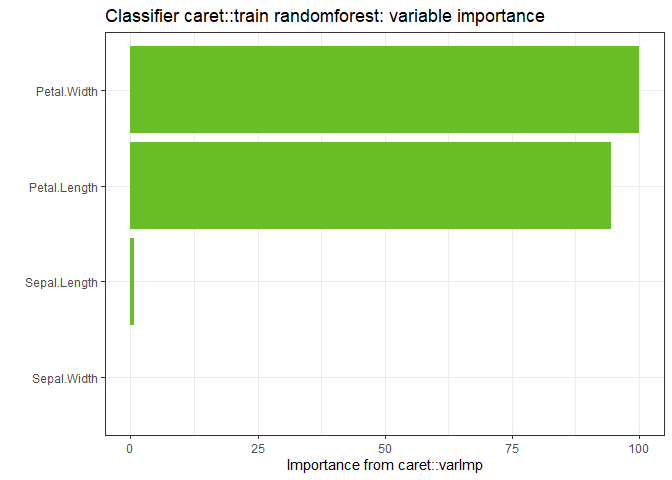## Overview

A tornado plot is a visualization of the range of outputs expected from a variety of inputs, or alternatively, the sensitivity of the output to the range of inputs. Tornado plots have a number of features:

• The center of the tornado is plotted at the response expected from the mean of each input variable.
• For a given variable, the width of the tornado is determined by the response to a change in each input variable while holding all others constant at their mean. Various criteria are used depending on the specifics of the analysis. Three primary criteria are implemented in this package:
• The range of the variable. The input is set at the maximum, minimum, and mean.
• A multiplicative factor of the variable mean. The input is set at $$100(1 - \alpha)$$% and $$100(1+\alpha)$$% of the mean.
• A quantile of the variable’s distribution in the input data. The input is set at $$100(1 - \alpha)$$ percentile and $$100(1+\alpha)$$ percentile
• Variables are ordered vertically with the widest bar at the top and narrowest at the bottom.
• Only one variable is moved from its mean value at a time.
• Factors or categorical variables have also been added to these plots by plotting dots at the resulting output as each factor is varied through all of its levels.
• The base factor level is chosen as the input variable for the center of the tornado when factors are present.

An importance plot attempts to dispay the relative impact of each variable on the model fit. Traditionally, for linear models, the concept of importance was expressed as the percentage of total response variable variance that is explained by each variable, either alone, or in the presence of the other variables in the model. Each model type can have different measures of importance. In this package, the following methods are used:

• Linear Models: percent of the total variance explained by each variable alone and cumulatively with other variables in the model.
• Generalized Linear Models: Same as linear models, but using the deviance instead of the variance.
• Survival Models, LASSO, Ridge Regression: The decrease in model Mean Squared Error as each variable is permuted in the data set. Permuting a varible in a data set remove the explanatory power of that variable relative to the other vriables in the model still correlated with the response variable.
• caret package models: The definition of variable importance for each model in the caret package is taken from the varImp method. See the caret package for specifics.

## Data Sets

A few standard data sets are used in these examples:

• mtcars: Motor Trend Car Road Tests - predict Miles Per Gallon (mpg) based on other car factors
• mtcars_w_factors: the mtcars dataset with a few numeric variables replaced with categorical or factor variables where appropriate. e.g. Automatic vs Manual transmission
• survival::ovarian: Ovarian Cancer Survival Data - predict survival time
• survival::bladder: Bladder Cancer recurrences - risk of bladder cancer recurrence
• iris: Edgar Anderson’s Iris Data - predict the type of iris from measurements
mtcars_w_factors <- mtcars
# Automatic vs Manual
mtcars_w_factors$am <- factor(mtcars$am)
# V or Straight cylinder arrangement
mtcars_w_factors$vs <- factor(mtcars$vs)
# number of cylinders
mtcars_w_factors$cyl <- factor(mtcars$cyl)
# number of forward gears
mtcars_w_factors$gear <- factor(mtcars$gear)
# number of carburetors
mtcars_w_factors$carb <- factor(mtcars$carb)

### LM

#### type = “PercentChange”

lm1 <- lm(mpg ~ cyl*wt*hp, data = mtcars)
plot(torn1, xlabel = "MPG", geom_bar_control = list(width = 0.4))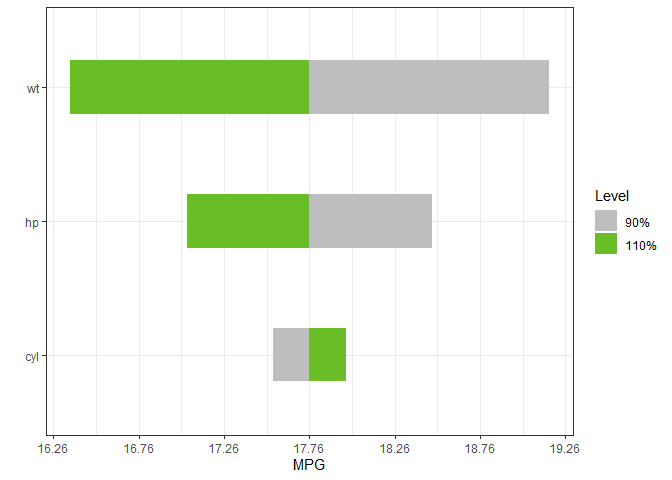#### type = “rangess”

torn2 <- tornado::tornado(lm1, type = "ranges")
plot(torn2, xlabel = "MPG", geom_bar_control = list(width = 0.4))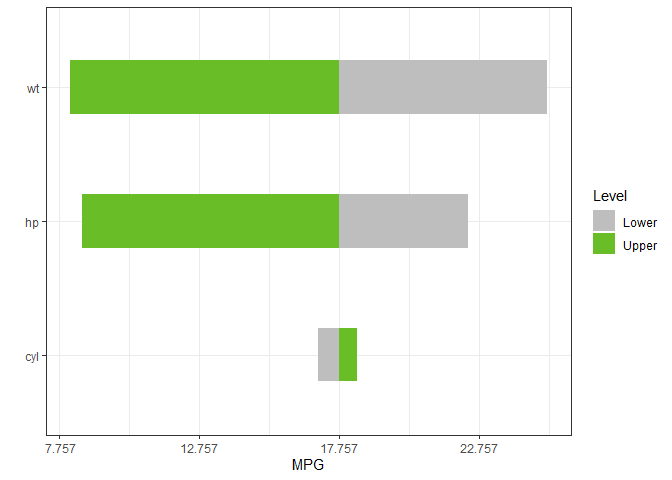#### type = “percentiles”

torn3 <- tornado::tornado(lm1, type = "percentiles", alpha = 0.05)
plot(torn3, xlabel = "MPG", geom_bar_control = list(width = 0.4))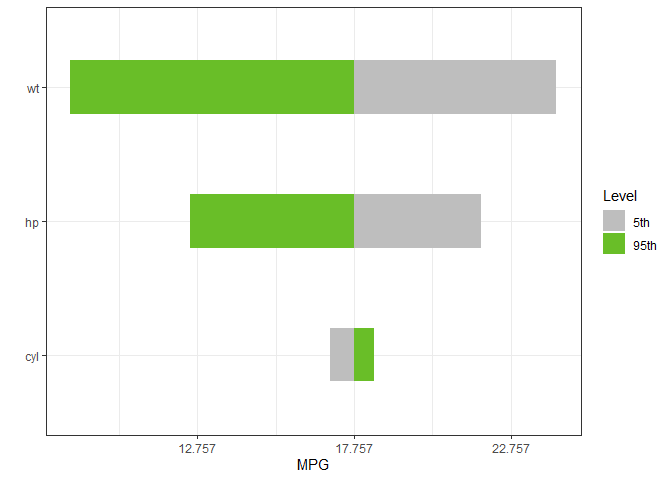#### Including Categorical Variables

lm4 <- lm(mpg ~ cyl + wt + hp + vs, data = mtcars_w_factors)
plot(torn4, xlabel = "MPG", geom_bar_control = list(width = 0.4))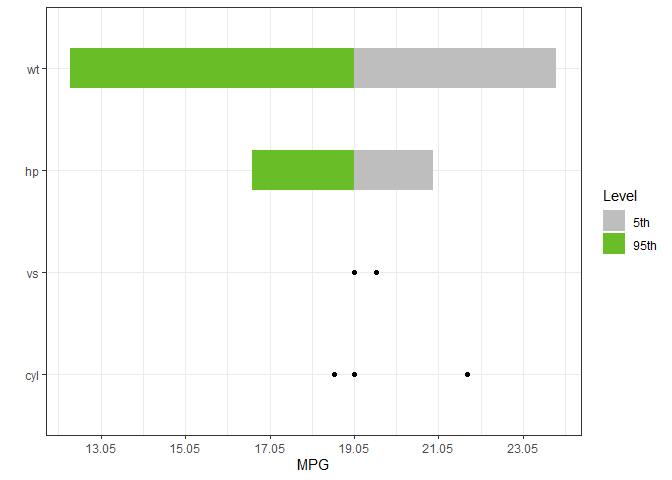#### Changing Variable Names

dict <- list(old = c("cyl", "wt", "hp", "vs"),
new = c("Cylinders", "Weight", "Horsepower", "V_or_Straight"))
torn5 <- tornado::tornado(lm4, type = "percentiles", alpha = 0.05, dict = dict)
plot(torn5, xlabel = "MPG", geom_bar_control = list(width = 0.4))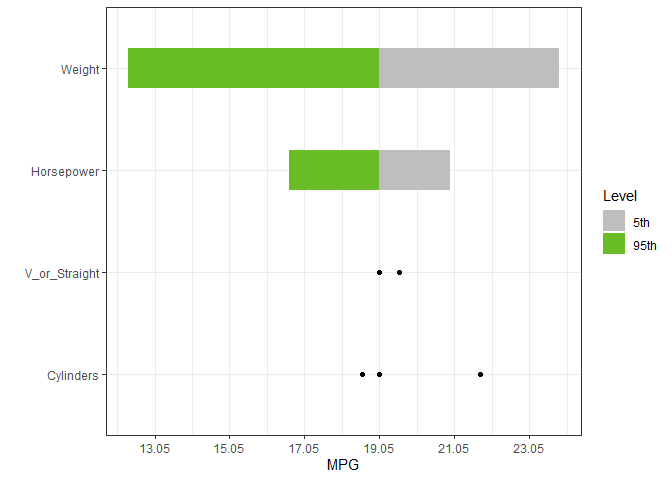#### Plotting Options

dict <- list(old = c("cyl", "wt", "hp", "vs"),
new = c("Cylinders", "Weight", "Horsepower", "V_or_Straight"))
plot(torn5, xlabel = "MPG", geom_bar_control = list(width = 0.4),
sensitivity_colors = c("#FC8D62", "#66C2A5"),
geom_point_control = list(size = 3, fill = "purple", col = "purple"))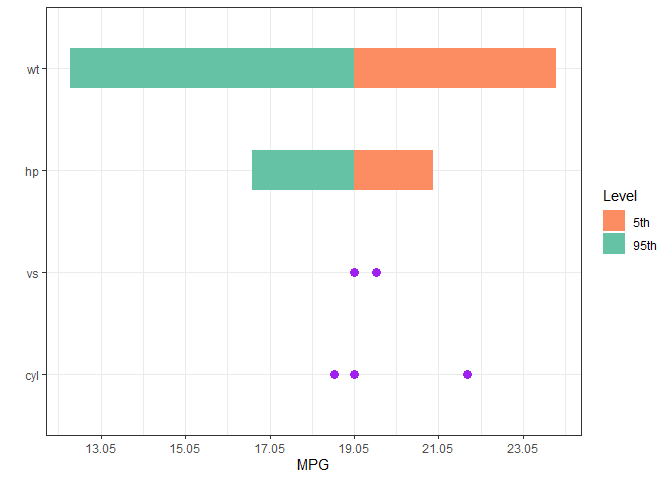#### Extending the ggplot

Notes:

• The plot center is at 0 and the x-axis is relabeled. So Adding lines to the plot should use a zero centered coordinate system
• Also, the plot is drawn using coord_flip, so the horizonal line below is draw vertically
g <- plot(torn5, plot = FALSE, xlabel = "MPG", geom_bar_control = list(width = 0.4),
sensitivity_colors = c("#FC8D62", "#66C2A5"),
geom_point_control = list(size = 3, fill = "purple", col = "purple"))
g <- g + ggtitle("Test Plot")
g <- g + geom_hline(yintercept = 0, col = "black", lwd = 2)
#> Warning: Using size aesthetic for lines was deprecated in ggplot2 3.4.0.
#> ℹ Please use linewidth instead.
plot(g)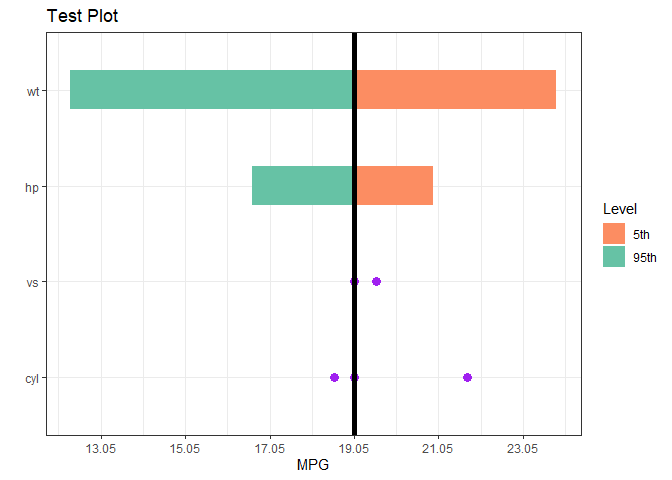### GLM

Predict if an engine is a V or Straight given covariates

glm1 <- glm(vs ~ wt + disp + cyl, data = mtcars, family = binomial(link = "logit"))
plot(torn1, xlabel = "V or Straight Engine", geom_bar_control = list(width = 0.4))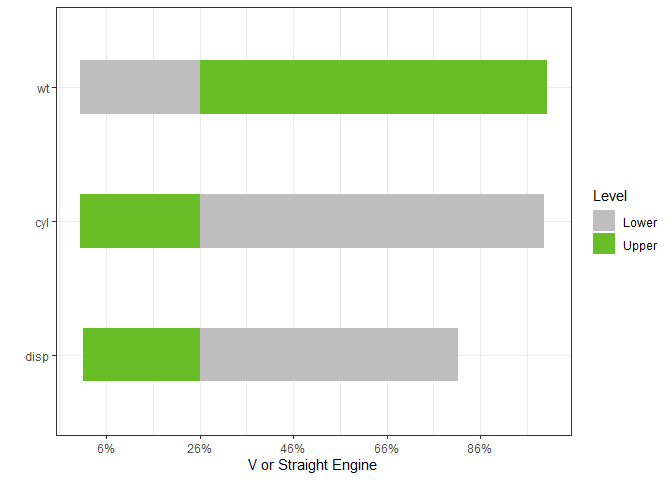### Censored Data

#### Surival Regression or Accelerated Failure

survreg1 <- survival::survreg(survival::Surv(futime, fustat) ~ ecog.ps + rx + age + resid.ds,
survival::ovarian, dist = 'weibull', scale = 1)
type = "PercentChange", alpha = 0.10)
plot(torn1, xlabel = "Survival Time", geom_bar_control = list(width = 0.4))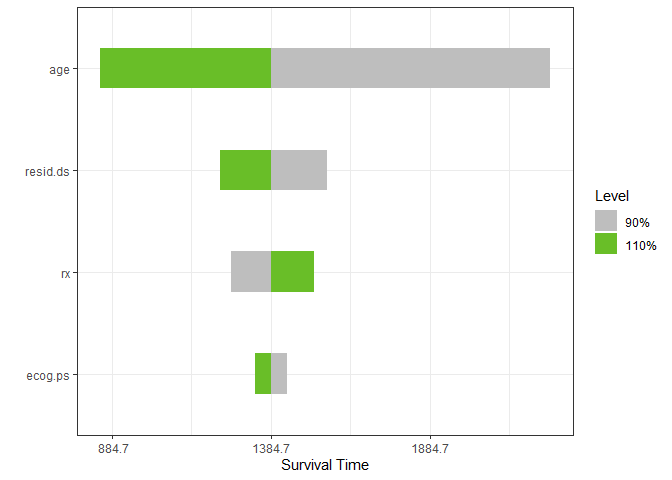#### Cox Proportional Hazards

coxph1 <- survival::coxph(survival::Surv(stop, event) ~ rx + size + number,
alpha = 0.10)
plot(torn1, xlabel = "Risk", geom_bar_control = list(width = 0.4))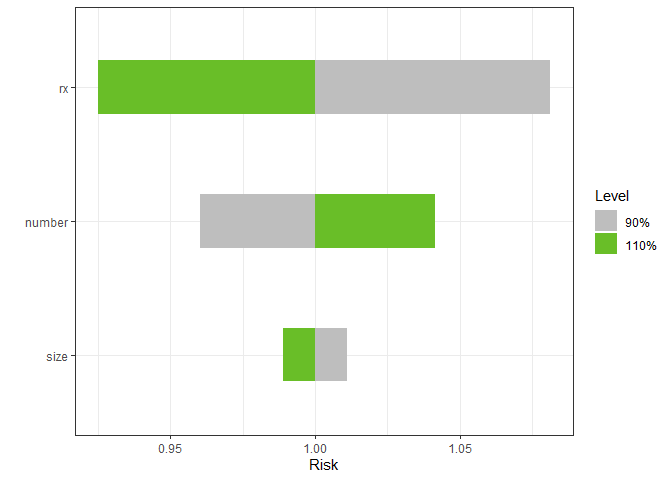### Ridge Regression and LASSO

if (has_glmnet)
{
form <- formula(mpg ~ cyl*wt*hp)
mf <- model.frame(form, data=mtcars)
mm <- model.matrix(form, mf)
gtest <- glmnet::cv.glmnet(x = mm, y = mtcars$mpg, family = "gaussian") torn <- tornado::tornado(gtest, modeldata = mtcars, form = formula(mpg ~ cyl*wt*hp), s = "lambda.1se", type = "PercentChange", alpha = 0.10) plot(torn, xlabel = "MPG", geom_bar_control = list(width = 0.4)) } else { print("glmnet is not available for vignette rendering") }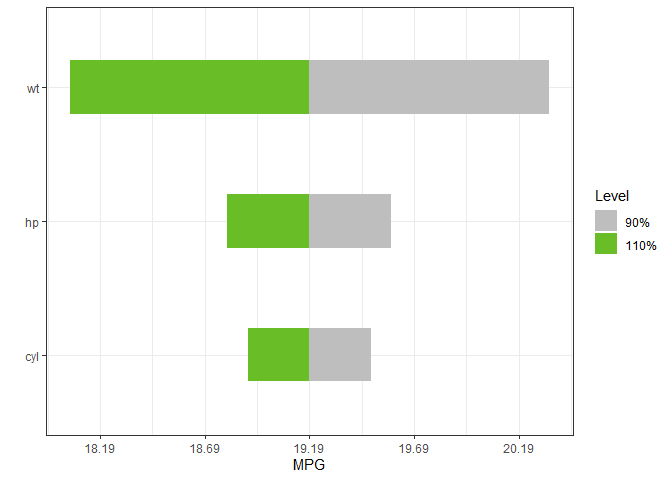### Machine Learning Models #### Regression if (has_caret) { gtest <- caret::train(x = subset(mtcars_w_factors, select = -mpg), y = mtcars_w_factors$mpg, method = "rf")
plot(torn, xlabel = "MPG")
} else
{
print("caret is not available for vignette rendering")
}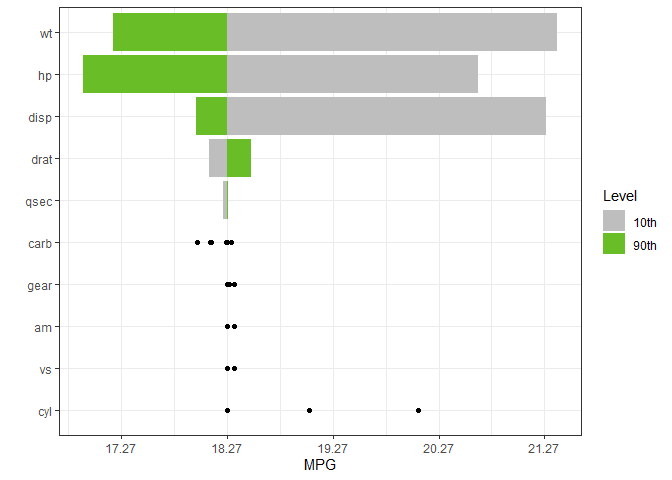#### Classification

The plot method can also return a ggplot object

if (has_caret)
{
gtest <- caret::train(x = subset(iris, select = -Species),
y = iris$Species, method = "rf") torn <- tornado::tornado(gtest, type = "percentiles", alpha = 0.10, class_number = 1) g <- plot(torn, plot = FALSE, xlabel = "Probability of the Setosa Species") g <- g + ggtitle("Classifier caret::train 'rf', 10th to 90th perc. of each var.") plot(g) torn <- tornado::tornado(gtest, type = "percentiles", alpha = 0.10, class_number = 2) g <- plot(torn, plot = FALSE, xlabel = "Probability of the versicolor Species") plot(g) } else { print("caret is not available for vignette rendering") }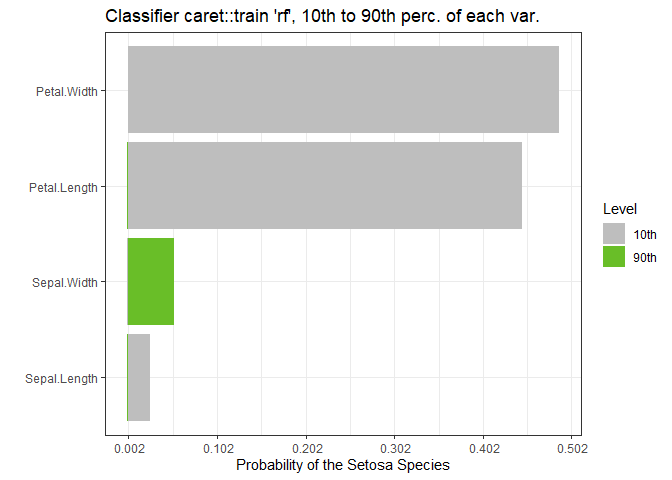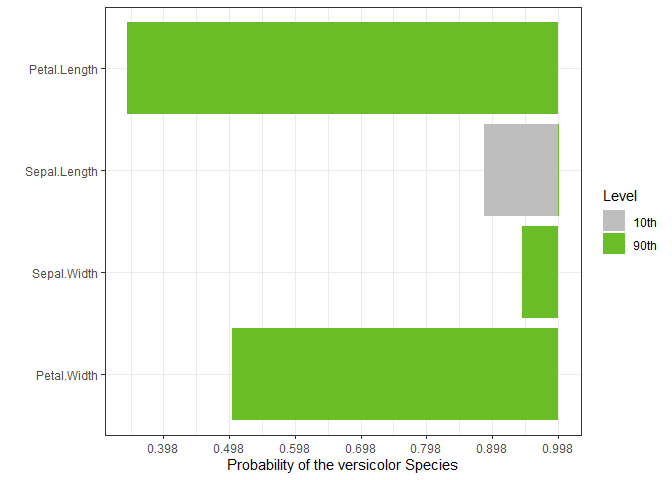## Importance Plots ### Linear Models gtest <- lm(mpg ~ cyl*wt*hp + gear + carb, data = mtcars) gtestreduced <- lm(mpg ~ 1, data = mtcars) imp <- tornado::importance(gtest, gtestreduced) plot(imp)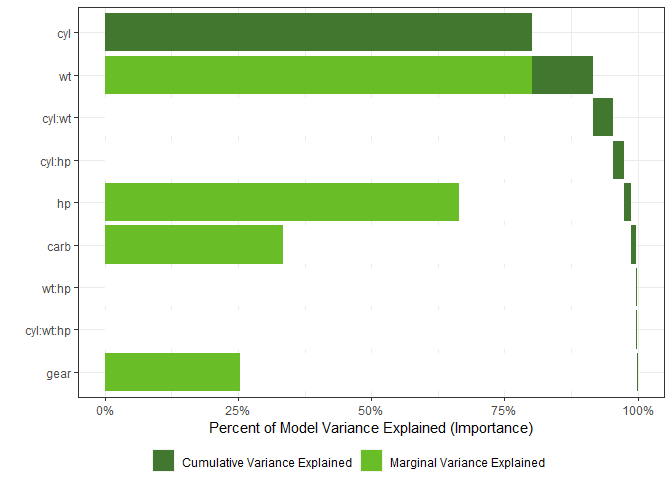#### Using a dictionary to translate the variable names and chaning colors dict <- list(old = c("cyl", "wt", "hp", "vs", "gear", "carb"), new = c("Cylinders", "Weight", "Horsepower", "V_or_Straight", "Num Gears", "Num Carbs")) imp <- tornado::importance(gtest, gtestreduced, dict = dict) plot(imp, col_importance_alone = "#8DD3C7", col_importance_cumulative = "#FFFFB3")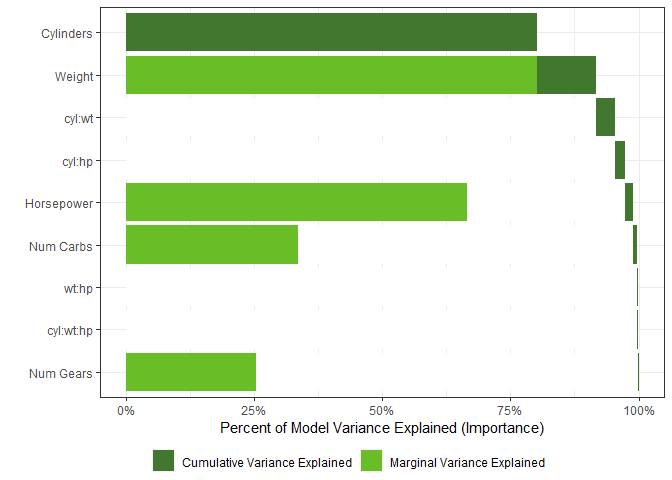### Generalized Linear Models gtest <- glm(vs ~ wt + disp + gear, data = mtcars, family = binomial(link = "logit")) gtestreduced <- glm(vs ~ 1, data = mtcars, family = binomial(link = "logit")) imp <- tornado::importance(gtest, gtestreduced) plot(imp)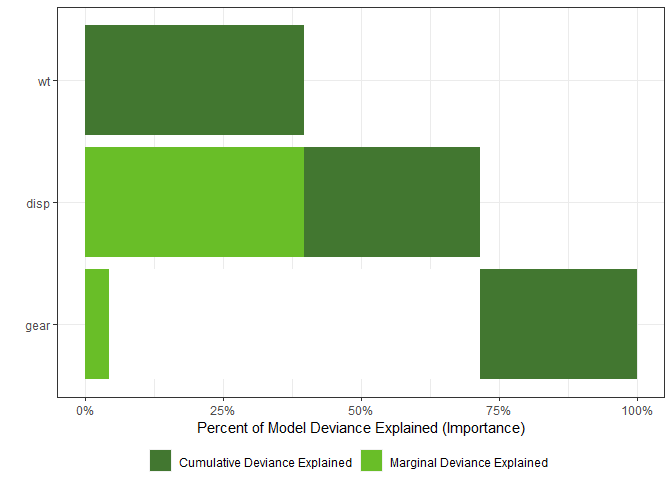### Censored Data model_final <- survival::survreg(survival::Surv(futime, fustat) ~ ecog.ps*rx + age, data = survival::ovarian, dist = "weibull") imp <- tornado::importance(model_final, survival::ovarian, nperm = 100) plot(imp, geom_bar_control = list(width = 0.4, fill = "blue"))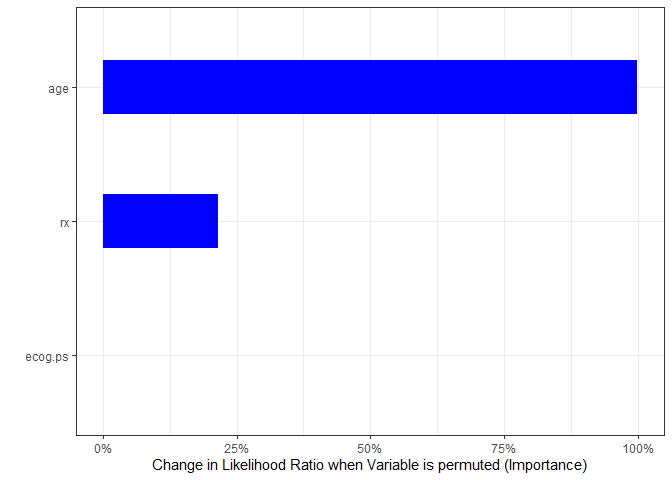### Machine Learning #### Regression The number of variables plotted can also be controlled if (has_caret) { gtest <- caret::train(x = subset(mtcars_w_factors, select = -mpg), y = mtcars_w_factors$mpg, method = "rf")
plot(imp, nvar = 7)
} else
{
print("caret is not available for vignette rendering")
}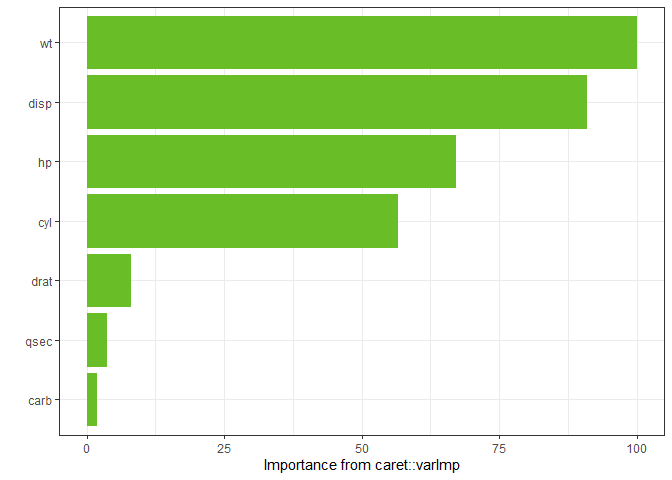#### Classification

The plot method can also return a ggplot object

if (has_caret)
{
gtest <- caret::train(x = subset(iris, select = -Species),
y = iris\$Species, method = "rf")
}